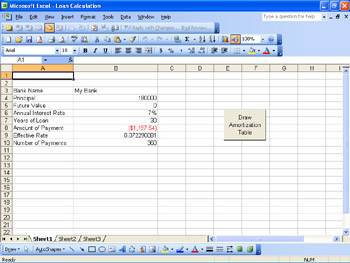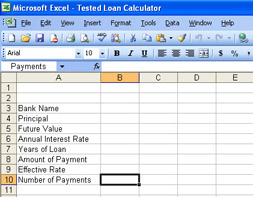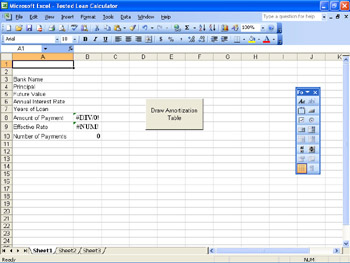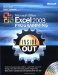# Referring to Ranges

 < Day Day Up >

The versatility of ranges is manifested in the number of ways you can refer to a range. Whether directly by cell address, as an offset from another cell, by name, or by using the current selection, referring to a range has many useful options. The Range property allows several methods of referring to a range. The syntax for the Range property is

` expression.Range(Cell1, Cell2)`

expression is a required element that should return either an Application, a Range, or a Worksheet object. If expression is omitted, ActiveSheet is implied.

Cell1 is a required variant that supplies the A1-reference to a cell or range of cells. If you use the Cell1 parameter to refer to a range of cells, the variant can include the range operator (a colon), the intersection operator (a space) or the union operator (a comma). Dollar signs (\$) can be included, but they are ignored. A locally defined name can also be supplied.

Cell2 is an optional variant that, when paired with Cell1, specifies the cell in the lower-right corner of the range.

As the property description implies, there are many ways you can specify which cells to include. You can use absolute references by using the ActiveSheet as the base object (expression), or you can use relative references by using the ActiveCell or other range object as the base object. You can specify a single cell, a group of continuous cells, a group of intermittent cells, or entire rows or columns.

### Referencing Ranges on the Active Worksheet

Referring to a range on the active worksheet is the most common method, and the majority of range references in VBA are geared toward using the active worksheet. You can refer to a range on the ActiveSheet by simply using the Range property. For example, to refer to cell D6 on the active worksheet, you could use the following code: Range("D6").Select.Inside Out
Staying Away from Select

Although the tendency is to always select a range first, it's not necessary to do so and it will put a performance hit on your procedure. (See 'Copying Data Between Ranges and Arrays' later in this chapter for more information.) If you have to use only a single formatting command, specify the range and the formatting all in one line, rather than selecting the range first and then doing the formatting.

As an example, to highlight the entire list of 6:00 P.M. entries in the Y2001ByMonth.xls workbook and make the font color blue, you would use the following command:

` Range("M6:M36").Font.ColorIndex = 41 `### Referencing Ranges on an Inactive Worksheet

The number of worksheets in an Excel workbook is limited only by the amount of available memory. Information can be spread across any number of worksheets, not just the active worksheet. To reference a range on an inactive worksheet, you need to specify the worksheet that contains the range.

Using the Range property on an inactive worksheet is no different from using it on the ActiveSheet. When the worksheet object is not specified, the active worksheet in implied. Going back to previous example of reference cell D6, on an inactive worksheet it would be referenced using code similar to the following:

`Worksheets(2).Range("D6").Select.`

It is possible to reference a range on an inactive worksheet without specifying the worksheet, but only if the range is named. (See 'Using Named Ranges' later in this chapter.) By using the statement Range("Frequency").Select the named range Frequency is selected, regardless of whether it is on the active worksheet or not.

### Referencing Cells in a Range

Referencing an individual cell within a range works the same as referencing a cell within the entire workbook. The cell in the top-left corner of the range would be addressed as 'A1'. The cell three rows down and four columns to the right would be cell 'D3'. As an example, in the workbook Y2001ByMonth.xls, you could assign the range D6:O36 to a range object. To reference the 9:00 A.M. entry on the first day (cell D6 in the worksheet), you would use cell A1 of the range object. Likewise, the 7:00 P.M. of the sixteenth day (cell N21 in the worksheet) would be cell K16 of the range object.

### Referencing Cells Using the Offset Property

If the information you need to use is located in a particular location away from a known cell, you can use the Offset property to reference the cell. By specifying the number of rows and columns from a set location, you can reference the cell. It's similar to giving driving directions where you tell someone to start at a particular location. The person you're giving directions to needs to get to the starting spot on their own, but once there, they can follow your directions to reach the final destination.

The Offset property works by moving the number of rows and columns specified. Positive numbers move down and to the right while negative numbers move up and to the left. A zero maintains the current row or column. The Calculate_Table routine inside the Loan Calculation.xls workbook, shown in Figure 8-1, uses the Offset property to set the formula for the Present Value and Interest Paid columns.Figure 8-1: This sample worksheet lets you calculate the components of a mortgage loan.

`Sub Calculate_Table()Dim counter As Integer, Payments As Integer, Frequency As IntegerDim Temp As String, intRow As Integer, intColumn As IntegerDim intOffset As Integer, strR1C1Address As String, strA1Address As String     ActiveWorkbook.Sheets.Add After:=Worksheets(Worksheets.Count)    Sheets(Sheets.Count).Select    ActiveSheet.Name = Range("Name")    Payments = Range("Payments")    Frequency = 12       ' Set headers    Range("A5") = "Payment"    Range("B5") = "Principal"    Range("C5") = "Interest"    Range("D5") = "Present Value"    Range("E5") = "Interest Paid"        ' Loop through number of payments and insert formulas    ' Use various methods of referencing a range    For counter = 1 To Payments        intRow = 5 + counter        intColumn = 1        strR1C1Address = "R" & Format(intRow, "#0") & "C" & _            Format(intColumn, "#0")        strA1Address = Application.ConvertFormula(strR1C1Address, _            xlR1C1, xlA1)        Range(strA1Address) = "Payment:" + Str\$(counter)        Range(strA1Address).Range("B1") = "=PPMT(APR/" & _            Str\$(Frequency) & "," & Str\$(counter) & _            ",Payments,Principal, Future_Value)"        Range(strA1Address).Select        Selection.Range("C1") = "=IPMT(APR/" & Str\$(Frequency) _            & "," & Str\$(counter) & ",Payments,Principal," _            & " Future_Value)"        Selection.Offset(0, 3) = "=R[-1]C+RC[-2]"        Selection.Offset(0, 4) = "=RC[-2]+r[-1]c"    Next counter        ' Reset "Present Value" and "Interest Paid" formulas for     ' first payment    Range("D6").Formula = "=Principal+B6"    Range("E6").Formula = "=C6"        ' Autosize columns to necessary width    Columns("A:E").Select    Columns.EntireColumn.AutoFit        ' Set Titles    Range("A1").Value = ActiveSheet.Name    Range("A1:E1").Select    Selection.Merge    Range("A2").Value = "Amortization Chart"    Range("A2:E2").Merge    With Range("A1")        .HorizontalAlignment = xlCenter        .Font.Bold = True    End With    With Range("A2")        .HorizontalAlignment = xlCenter        .Font.Bold = True    End With End Sub `Building a Version of the Loan Calculation Workbook

The Calculate_Table macro relies upon several features in Excel to work, such as named ranges (discussed later in this chapter), form controls, and command buttons. To build the workbook yourself, follow these steps.

1. Open a blank workbook by clicking the New button on the Standard toolbar or selecting Blank workbook from the New Workbook Task Pane.

2. In column A, type the following descriptions, one per line. You can use Figure 8-2 as a guide:

• Bank Name

• Principal

• Future Value

• Annual Interest Rate

• Years of Loan

• Amount of Payment

• Effective Rate

• Number of PaymentsFigure 8-2: The Loan Calculator requires a number of specific inputs-here are those descriptions.

3. In column B, assign names to the cells alongside the description. Match the cell name in column B to the description in column A: Name, Principal, Future_Value, APR, Years, Payment. Skip the cell for Effective Rate, and name the last cell Payments.

4. Select the field cell for Amount of Payment, and type the following formula: =PMT(APR/12,Payments,Principal, Future_Value)

 Note When you first enter this formula, a #DIV/0 error will appear in the cell because there are no values in the cells used in the formula. Once you enter those values, the error will disappear.

5. Select the field cell for Effective Rate, and type in the following formula: =EFFECT(APR,12)

 Note When you first enter this formula, a #NUM! error will appear in the cell because there is no value in the Years cell. Once you enter a value in that cell, the error will disappear.

6. Select the field cell for Number of Payments, and type the following formula: =Years*12

7. After you have typed in the Calculate_Table macro, select the Button button on the Forms tool bar and draw a button on the worksheet, as shown in Figure 8-3. The Assign Macro dialog box will appear, allowing you to choose the Calculate_Table macro.Figure 8-3: Adding the Button Control to the form.

8. With the button still selected, select the text in the button and type in the following: Draw Amortization Table.

Once you type information in the fields, you can click the Draw Amortization Table button to have the Calculate_Table macro executed. If you want to run the macro again, you need to change the name of your bank, which is used as the name of the new worksheet. If you try to create a worksheet with a duplicate name, the macro will generate an error.### Defining a Range Using the Cells Property

Another method of referencing the same cell would be to specify the cell using the Cells method. To do so, you would have to specify the row and column numbers of the cells in opposite corners of the range you want to specify. For a single cell, you have to specify the same cell twice; after all, it's the same cell in opposite corners. For example, to select cell D6, you would use the following code:

`Range(Cells(4,6), Cells(4,6)).Select`

### Referencing Columns and Rows

Referring to an entire column or row is done using the Columns or the Rows property. You can select a single column or row or multiple columns or rows. This code snippet from the Calculate_Table routine selects columns A through E and resizes them to fit the contents of the cells.

`Columns("A:E").SelectColumns.EntireColumn.AutoFit`

### Referencing Non-Contiguous Groups of Cells

Not all ranges you need to work with will be contiguous. You can specify non-contiguous cells by separating the ranges with commas. For example, to select all of the sales data for Mondays in January in the Y2001ByMonth.xls workbook, you could use the following code: Range("D6:O6, D13:O13, D20:O20, D27:O27, D34:O34").Select.

 < Day Day Up >Microsoft Office Excel 2003 Programming Inside Out (Inside Out (Microsoft))
ISBN: 0735619859
EAN: 2147483647
Year: 2006
Pages: 161

Similar book on Amazon

flylib.com © 2008-2017.
If you may any questions please contact us: flylib@qtcs.net